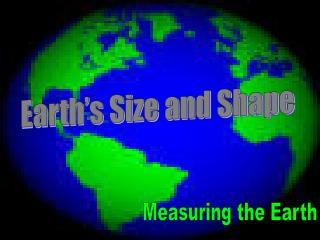DownloadDownload PresentationEarth’s Size and Shape

# Earth’s Size and Shape

Download Presentation## Earth’s Size and Shape

- - - - - - - - - - - - - - - - - - - - - - - - - - - E N D - - - - - - - - - - - - - - - - - - - - - - - - - - -
##### Presentation Transcript

1. Earth’s Size and Shape Measuring the Earth

2. Earth’s Shape • VERY CLOSE TO BEING A PERFECT SPHERE • OBLATE SPHEROID • FLATTENING AT THE POLAR REGIONS • SLIGHT BULGE AT THE EQUATOR

3. Therefore the equatorial diameter is larger

4. The Earth is very slightly oblate about a 43km difference between the polar and equatorial diameters

5. What proof is there that we are slightly oblate? • Gravity measurements. Gravity is the force of attraction between any 2 objects. Increase mass of objects = increase in gravity Decrease of distances = increase in gravity • If Earth were a perfect sphere, it would be expected to exert an equal force on objects at equal distances from the center of earth.

6. Weight!!! – measure of gravitational force • We are further from the center of the Earth at the equator – gravity is less • This means we weigh LESS!!! • We are closer at the poles… • We weigh MORE!!!!

7. The least amount of gravity is farther from the center of Earth High Mountains farther from the center of earth – gravity is less

8. The Earth appears to look like a sphere from space

9. The Oblate Sphere • The difference is small • We can not see it with the naked eye • It still appears like a sphere • Be careful on multiple choice

10. Why is the earth not perfectly round? • Earth’s rotation causes… Bulging at equator Flattening at the poles

11. EVIDENCE that Earth is round

12. EVIDENCE 1.) PHOTOGRAPHS FROM OUTER SPACE

13. EVIDENCE 2.) Ships appear to sink gradually below horizon

14. EVIDENCE 3.) SPHERICAL SHADOW CAST DURING AN ECLIPSE OF THE MOON

15. Lunar Eclipse • Only a sphere can cast a shadow that appears round. During a lunar eclipse, the earth casts its shadow on the moon during the full moon phase.

16. EVIDENCE 4.) GRAVITY IS NEARLY EQUAL STRENGTH AT SEA LEVEL ALL OVER THE EARTH’S SURFACE CAREFUL MEASUREMENTS SHOW THAT THERE IS A SLIGHTLY GREATER GRAVITATIONAL PULL AT THE POLES

17. What proof is there that we are slightly oblate? • Gravity measurements. Gravity is the force of attraction between any 2 objects. Increase mass of objects = increase gravity Decrease distances = increase gravity • If Earth were a perfect sphere, it would be expected to exert an equal force on objects at equal distances from the center of earth.

18. The least amount of gravity is farther from the center of Earth High Mountains farther from the center of earth – gravity is less

19. Why is the earth not perfectly round? • Earth’s rotation causes… Bulging at equator Flattening at the poles

20. EVIDENCE 5.) The angle of Polaris above the horizon would not change on a flat Earth.

21. Polaris Polaris North Star • This is the star that lies in space practically over the geographic North Pole of the earth. • If you stood at the North Pole, Polaris would be almost directly overhead.

22. Why do observations of Polaris help determine the Earth’s shape????? • The North Star appears lower and lower in the sky as you travel toward the equator because of earth’s spherical shape. • At the North Pole, Polaris appears directly overhead. • At the Equator, Polaris appears at the horizon. • Polaris cannot be seen below the Equator in the Southern Hemisphere.

23. Polaris Changes in Altitude – Polaris is a fixed point above the North Pole. 90º

24. Polaris – Fixed Point above the North Pole • Because of this, in the Northern Hemisphere, the altitude (angle measured in degrees above the horizon) of Polaris tells observer his latitude position. • If observer’s latitude changes in the Northern Hemisphere, the altitude of Polaris will exactly match observer’s latitude. ALT OF POLARIS = LAT OF OBSERVER

25. CIRCUMFERENCE OF THE EARTH ERATOSTHENES (TEXT PG. 19) CALCULATED THE CIRCUMFERENCE OF THE EARTH ABOUT 2000 YRS AGO

26. Erathosthenes • Made assumptions that… a. The earth is round b. The sun’s rays are parallel Using simple geometry he set up an equation that determined the circumference of the earth.

27. Syene Alexandria

28. How did he calculate it? 7.2º 360º 5000 stadia X = CIRCUMFERENCE = 250,000 stadia HIS CALCULATION WAS EQUIVALENT TO 46,250 KM

29. What was Erotasthenes Percent Deviation? • THE ACTUAL CIRCUMFERENCE IS ABOUT 40,000 KM ERATOSTHENES PERCENT DEVIATION WAS 16%

30. TOTAL SURFACE AREA 510 MILLION SQUARE KM • WATER = 361 MILLION SQUARE KM • LAND = 149 MILLION SQUARE KM

31. Earth's Layers EARTH IS MADE UP OF A SERIES OF SPHERES HELD TOGETHER BY GRAVITY ARRANGED FROM LOWEST TO HIGHEST DENSITY

32. ATMOSPHERE THE LAYER OF GASES THAT SURROUNDS THE EARTH ABOVE THE SURFACE page 14 in ESRT • COMPOSED MAINLY OF NITROGEN AND OXYGEN • HUNDREDS OF KM THICK • FARTHEST FROM THE EARTH’S CENTER BECAUSE IT IS THE LEAST DENSE • STRATIFIED INTO LAYERS OR ZONES EACH WITH ITS OWN DISTINCT CHARACTERISTICS • TROPOSPHERE, STRATOSPHERE, MESOSPHERE, • THERMOSPHERE • THE BOUNDARIES BETWEEN LAYERS ARE • CALLED PAUSES

33. HYDROSPHERE THE LAYER OF LIQUID WATER THAT LIES BETWEEN THE ATMOSPHERE AND MOST OF THE LITHOSPHERE • COVERS 71% OF THE EARTH’S SURFACE • AVE 3.8 KM IN THICKNESS • MOSTLY HYDROGEN AND OXYGEN

34. LITHOSPHERE LAYER OF ROCK WHICH FORMS THE SOLID OUTER SHELL • APPROX. 100 KM THICK • DIVIDED INTO LITHOSPHERIC PLATES